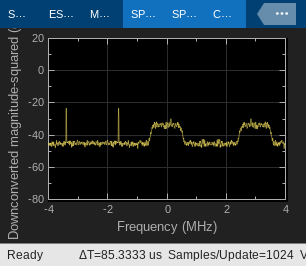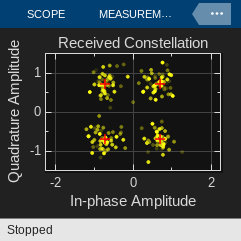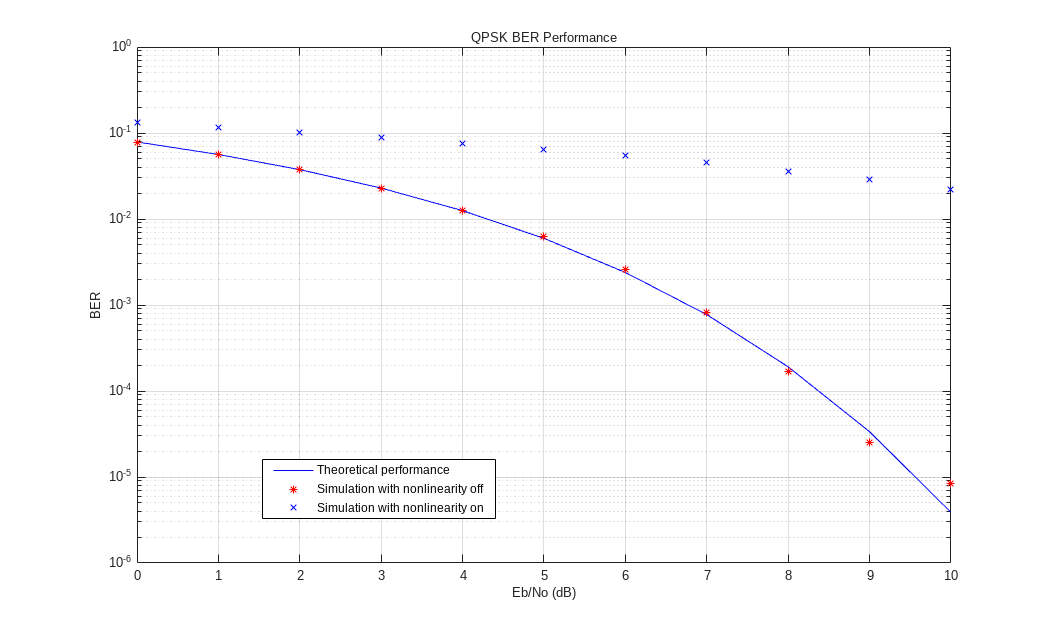# Passband Modulation

This model shows a straightforward way to perform passband modulation, by multiplying a modulated complex signal with a complex sine wave to perform frequency upconversion. In general, it is preferable to model a system at complex baseband. However, there are some circumstances where it is necessary to model the system at real passband. An example of this is when an adjacent band signal is processed with a nonlinearity, and causes interference in the desired band. This model also illustrates the effect of such interference.### Structure of the Example

The communications link in this model includes these components:

• A `Random Integer Generator` block, used as source of random data

• A modulator and a pulse shaping filter that perform QPSK modulation and root raised cosine pulse shaping.

• An `Upconverter` block that multiplies the modulated signal by a carrier frequency.

• A source of tone interference. The interference has a cubic nonlinearity which may be toggled on or off. When the nonlinearity is off, the interference falls completely out of band, but when on, the third harmonic of the tone is introduced into the desired band, causing co-channel interference.

• An `AWGN Channel` block, set to Eb/No mode. It specifies two bits per symbol because the modulation format is QPSK. The signal power is 1/(2*8) watts. This is because the original signal power at the modulator is 1 watt. The root-raised cosine filter upsamples the signal by a factor of 8, which reduces the power by that factor. The frequency upconversion block output takes only the real part of the signal, thereby reducing the power again, this time by a factor of 2. Finally, the symbol period is 1e-6 seconds, to match the original sample time on the `Random Integer Generator` source.

• A `Downconverter` block that converts the signal from real passband to complex baseband.

• A root raised cosine pulse shaping filter that decimates back to one sample per symbol, and a QPSK demodulator block.

• BER and RMS EVM metric calculation blocks.

### Results and Displays

When the simulation runs, two spectrum analyzers and one scatter plot open.

The first spectrum analyzer shows the signal and the interference signal at passband. With the nonlinearity turned off, the spectrum of the tone interferer falls outside the bandwidth of the desired signal. With the cubic nonlinearity on, the third harmonic of the interference falls into the band of the desired signal.The second scope illustrates the signal after it has been downconverted back to baseband at the receiver, prior to the root raised cosine filtering. Note that with the nonlinearity on, you can see the interfering tone present with the baseband signal.The third scope shows the scatter plot of the received signal, and by toggling the nonlinearity on and off, you can view the effect the interference has on the scatter plot. With the nonlinearity on, the signal constellation is more diffuse than when the nonlinearity is not present.The model also contains two numerical displays. The first one displays the BER of the link. The BER calculation resets each time the nonlinearity is toggled on or off.

The second numerical display is the RMS Error Vector Magnitude (EVM) measured with the EVM Measurement block.

### Experimenting with the Example

Double-click on the `Nonlinearity on/off` block to toggle the nonlinearity on the interference signal. Observe the changes this has on the received spectrum, constellation, BER and EVM.

By varying the Eb/No parameter, you can produce BER curves, and compare the results of the model with theoretical results. Note that the model achieves expected theoretical results[ 1 ] for QPSK with the nonlinearity off. Furthermore, you can see the effects the nonlinearity has on overall BER.

For further experimentation, try changing the value of the Eb/No parameter on the AWGN channel block, or changing the power of the interference signal. To change the power of the interference signal, open the `Interference with Nonlinearity` subsystem, and modify the gain value.The `Downconverter` block uses a simple complex multiplication method to perform downconversion. You can find an example showing more efficient downconversion using IF subsampling at: IF Subsampling with Complex Multirate Filters